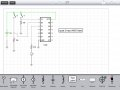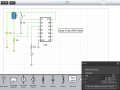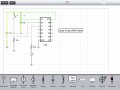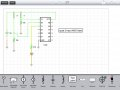# First Electrical Engineering Lab, Need Help!

Thread Starter

#### Hunter Neumann

Joined Aug 24, 2015
53
Today in my first electrical engineering lab we used the chips 7404, 7408, and 7432 to perform the equation
P(x,y,z) = x ˑ(y + z) + y’ˑz. We put the chips on to a breadboard which was connected to a C.A.D.E.T Testing system. (I just bought one for myself for some home practice, not sure how useful it will be but I want to make sure I have the resources I need to understand all of this stuff) But any way, I guess I should provide some background info on myself before I get into the problems I'm having. I am a freshman in college and this is my second week in the Electrical Engineering program at my school. I consider myself to be smart, but not crazy smart. The terminology I use is probably going to be off so I would love some feedback critiquing what I say. So far we have only covered the basics. Not, And, and Or Gates as well as corresponding truth tables and diagrams of Basic Boolean Algebraic equations. I get how to do all of this on paper but when it comes to implementing it on the breadboard, switches, and Led I'm a little bit lost. I was with a guy (my partner in lab) that had tons of experience wiring cars so he went through it without any issues however I didn't learn very much. I get how to connect the individual chips to power and ground but when implementing the equation onto the board I'm a little lost. I will be uploading pictures of the lab a little later if it would help you guys help me. Im not really sure how much information I should be telling you or if I'm missing something important. So anyway, all I'm asking is how to implement the variables (switches) to the three chips and how to carry out the equation between them to where the LED responds according to what the output of the equation should be. Thanks for your help and if you need any clarification on anything to help me out please ask and ill make sure to respond as fast as I can.

#### GopherT

Joined Nov 23, 2012
8,012
Today in my first electrical engineering lab we used the chips 7404, 7408, and 7432 to perform the equation
P(x,y,z) = x ˑ(y + z) + y’ˑz. We put the chips on to a breadboard which was connected to a C.A.D.E.T Testing system. (I just bought one for myself for some home practice, not sure how useful it will be but I want to make sure I have the resources I need to understand all of this stuff) But any way, I guess I should provide some background info on myself before I get into the problems I'm having. I am a freshman in college and this is my second week in the Electrical Engineering program at my school. I consider myself to be smart, but not crazy smart. The terminology I use is probably going to be off so I would love some feedback critiquing what I say. So far we have only covered the basics. Not, And, and Or Gates as well as corresponding truth tables and diagrams of Basic Boolean Algebraic equations. I get how to do all of this on paper but when it comes to implementing it on the breadboard, switches, and Led I'm a little bit lost. I was with a guy (my partner in lab) that had tons of experience wiring cars so he went through it without any issues however I didn't learn very much. I get how to connect the individual chips to power and ground but when implementing the equation onto the board I'm a little lost. I will be uploading pictures of the lab a little later if it would help you guys help me. Im not really sure how much information I should be telling you or if I'm missing something important. So anyway, all I'm asking is how to implement the variables (switches) to the three chips and how to carry out the equation between them to where the LED responds according to what the output of the equation should be. Thanks for your help and if you need any clarification on anything to help me out please ask and ill make sure to respond as fast as I can.
Welcome, Hunter. You should ask the Moderator in duty to move your question to the Homework section. People there will guide you through - but you need to show some attempt to get thud solved (wrong or right, get started).

Thread Starter

#### Hunter Neumann

Joined Aug 24, 2015
53
Welcome, Hunter. You should ask the Moderator in duty to move your question to the Homework section. People there will guide you through - but you need to show some attempt to get thud solved (wrong or right, get started).

How do I do that? I have no problem with a moderator moving it, I thought this was the best spot for the question. As far as showing an attempt to solve the equation I will be uploading the truth tables and circuit diagram of the equation that I have completed later tonight or this weekend. I can easily do all of it on paper but I didn't get to learn much about the hands on stuff such as using the wires to connect from pin to pin and gate to gate to solve the equation. Thanks for the response

#### Papabravo

Joined Feb 24, 2006
16,182
In the algebra of real numbers you learned how to break down a complicated expression by by starting with the expressions inside of parentheses. You also may remember that multiplication should be done before addition. In Boolean algebra we have only the two elements 0 and 1, and the operations of AND (similar to multiplication) and OR (similar to addition). You also have NOT or inversion which has no analog in the algebra of real numbers. If you count the operations in your expression, you have two AND operations and two OR operations and one NOT operation. Each operation has two inputs and the physical gates each have two inputs. In the three chips you mentioned there are six inverters, four 2-input AND gates, and four 2-input OR gates. You should be able to implement this function with gates to spare in each of the packages. I look forward to your solution.

Thread Starter

#### Hunter Neumann

Joined Aug 24, 2015
53
•Russmax
Thread Starter

#### Hunter Neumann

Joined Aug 24, 2015
53
In the algebra of real numbers you learned how to break down a complicated expression by by starting with the expressions inside of parentheses. You also may remember that multiplication should be done before addition. In Boolean algebra we have only the two elements 0 and 1, and the operations of AND (similar to multiplication) and OR (similar to addition). You also have NOT or inversion which has no analog in the algebra of real numbers. If you count the operations in your expression, you have two AND operations and two OR operations and one NOT operation. Each operation has two inputs and the physical gates each have two inputs. In the three chips you mentioned there are six inverters, four 2-input AND gates, and four 2-input OR gates. You should be able to implement this function with gates to spare in each of the packages. I look forward to your solution.
I posted the lab above

#### DerStrom8

Joined Feb 20, 2011
2,390
Hello Hunter, welcome to AAC!

Just a couple of notes:

X + Y : This means X OR Y. In other words, X is put on one input pin of an OR gate, and Y is put on the other input. X + Y is on the output.
XY: This means X AND Y. In other words, X is put on one input of an AND gate, and Y is put on the other input. XY is on the output
X': This means NOT X. In other words, X is put on the input of a NOT gate. X' is on the output.

Hope this helps a bit!
Matt

#### Papabravo

Joined Feb 24, 2006
16,182
I posted the lab above
I didn't see the explicit use of an inverter in your diagram. Each of the symbols looked like a 2-input gate which must be wrong. A freehand drawing is just about the worst way to make a logic schematic. You need to use a drawing program or at least get a template for the hand drawn schematics, and make them large and well organized so it is possible to follow the logic.

Edit: I went back and looked again at your drawing and found the inverter.

Sugggetion:
LTSpiceIV is a free download from Linear Technology. Besides using it as a simulator, it has an adequate schematic drawing package that can be used for quick schematic interchange.

Last edited:
Thread Starter

#### Hunter Neumann

Joined Aug 24, 2015
53
I didn't see the explicit use of an inverter in your diagram. Each of the symbols looked like a 2-input gate which must be wrong. A freehand drawing is just about the worst way to make a logic schematic. You need to use a drawing program or at least get a template for the hand drawn schematics, and make them large and well organized so it is possible to follow the logic.

Edit: I went back and looked again at your drawing and found the inverter.

Sugggetion:
LTSpiceIV is a free download from Linear Technology. Besides using it as a simulator, it has an adequate schematic drawing package that can be used for quick schematic interchange.
Due to my professor I had no choice but to handwrite this lab, I can see how a simulator would really help out. Im downloading it right now to check it out

#### shteii01

Joined Feb 19, 2010
4,644
Yeah, your writing will need a lot of work. Don't be surprised if your early lab reports will have a lot of red.

•Hunter Neumann
Thread Starter

#### Hunter Neumann

Joined Aug 24, 2015
53
these aren't due until next Wednesday, do you have any suggestions on what I could change?

Thread Starter

#### Hunter Neumann

Joined Aug 24, 2015
53
Yeah, your writing will need a lot of work. Don't be surprised if your early lab reports will have a lot of red.
met to reply, see post above^^

Thread Starter

#### Hunter Neumann

Joined Aug 24, 2015
53
okay so this is a diagram on ICircuit of just the basics so far, I'm going to attempt to diagram the rest of the equation this weekend. Just looking on some feedback of what I have so far and how to put the led and switches using Icircuit. Thanks for your time. I also ordered wires, 7400, 7402, 7404, 7408, and 7432 chips to try on my own CADET system I ordered earlier in the week. Any certain type of wire I should have gotten? I just got a jumper wire cable kit for solderless breadboard. All feedback is appreciated

#### MrAl

Joined Jun 17, 2014
8,259
Hello there,

Perhaps the only thing missing from your understanding of going from equation to circuit is the concept of what is known as a *cascade* of devices.

When we cascade two devices that means we connect the output of the first device to the input of the second device. The input of the first device then becomes the main input, and the output of the second device then becomes the main output. So we end up with one input and one output, but we've connected two devices in cascade to achieve this result.

You seem to already know that the addition sign in boolean logic means "OR" and the multiplication sign (or no sign at all) means "AND". The single apostrophe signifies logical inversion, the "NOT" function.

With all this in mind, lets consider a simple expression like D=(A+B)*C.
Here we have two logical operators, OR and AND. We first OR the A and B inputs, then AND that result with the C input. That gives us an output we are calling D.
The cascade then is made from the OR gate followed by the AND gate. The output from the OR gate goes to one input of the AND gate (cascade) and the other input C goes to the other input of the AND gate. Thus we need a two input OR gate and a two input AND gate.

Inversion is almost the same. Say we have D=(A+B)'*C.
We still need the OR gate, but because now the output of the OR gate is inverted, we need to place an inverter at the output so that the output of the OR gate goes to the input of the NOT gate (inverter). The output is then inverted logically, and then that new output from the NOT gate goes to the input of the AND gate, and then again the third input C goes to the other input of the AND gate.

That was an example of a direct implementation, although we could apply some boolean logic first before we choose the gates. This helps reduce the complexity sometimes.

To try to reduce that last expression D=(A+B)'*C we could try applying the inversion first:
D=A'*B'*C

and now we have a single AND gate with inputs A', B', and C. We would need two inverters for A and B inputs unless we already had the inverted signals available in the system already. If we did have them then all we would need is a single three input AND gate.

You could try a couple simpler examples first before doing your assignment and we could check them here. For example, D=A*B+C. Can you find the gate arrangement for this expression?

Thread Starter

#### Hunter Neumann

Joined Aug 24, 2015
53
Hello there,

Perhaps the only thing missing from your understanding of going from equation to circuit is the concept of what is known as a *cascade* of devices.

When we cascade two devices that means we connect the output of the first device to the input of the second device. The input of the first device then becomes the main input, and the output of the second device then becomes the main output. So we end up with one input and one output, but we've connected two devices in cascade to achieve this result.

You seem to already know that the addition sign in boolean logic means "OR" and the multiplication sign (or no sign at all) means "AND". The single apostrophe signifies logical inversion, the "NOT" function.

With all this in mind, lets consider a simple expression like D=(A+B)*C.
Here we have two logical operators, OR and AND. We first OR the A and B inputs, then AND that result with the C input. That gives us an output we are calling D.
The cascade then is made from the OR gate followed by the AND gate. The output from the OR gate goes to one input of the AND gate (cascade) and the other input C goes to the other input of the AND gate. Thus we need a two input OR gate and a two input AND gate.

Inversion is almost the same. Say we have D=(A+B)'*C.
We still need the OR gate, but because now the output of the OR gate is inverted, we need to place an inverter at the output so that the output of the OR gate goes to the input of the NOT gate (inverter). The output is then inverted logically, and then that new output from the NOT gate goes to the input of the AND gate, and then again the third input C goes to the other input of the AND gate.

That was an example of a direct implementation, although we could apply some boolean logic first before we choose the gates. This helps reduce the complexity sometimes.

To try to reduce that last expression D=(A+B)'*C we could try applying the inversion first:
D=A'*B'*C

and now we have a single AND gate with inputs A', B', and C. We would need two inverters for A and B inputs unless we already had the inverted signals available in the system already. If we did have them then all we would need is a single three input AND gate.

You could try a couple simpler examples first before doing your assignment and we could check them here. For example, D=A*B+C. Can you find the gate arrangement for this expression?
I will try to diagram the equation you gave me tomorrow, thanks for your response. I tried to clean up my diagram of the equation we used in the lab by using Icircuit but I know its not right. Im not sure what to use for the LED or the variable switches or if I connected them wrong. Im new to the app so I'm not quite sure how it all works. I just used the analog switch and labeled them as the variables. I also used the lamp as my LED and tried to connect everything together to get the right output. Would love some feedback.

#### GopherT

Joined Nov 23, 2012
8,012
Hunter, your drawings are kind of a mess.

Also, your X, Y and Z are not set up correctly.

Here is an attempt to help you walk before you run. A single AND gate set up with two switches. Note, the way the switches are set up. When open (not connected) they are connected only to ground (through a 10k resistor), (a logic 0). When the switch is closed, the point is connected to +5V (logic 1).

I have all four combinations of switches. Note, the simulator will light the LED when the gate's output is high.•Hunter Neumann
Thread Starter

#### Hunter Neumann

Joined Aug 24, 2015
53
Hunter, your drawings are kind of a mess.

Also, your X, Y and Z are not set up correctly.

Here is an attempt to help you walk before you run. A single AND gate set up with two switches. Note, the way the switches are set up. When open (not connected) they are connected only to ground (through a 10k resistor), (a logic 0). When the switch is closed, the point is connected to +5V (logic 1).

I have all four combinations of switches. Note, the simulator will light the LED when the gate's output is high.
View attachment 91069

View attachment 91070

View attachment 91071

View attachment 91072
I guess I am getting ahead of myself trying to implement my lab equation onto ICircuit. I have modified it to look like this(see picture below) but I am going to save it at that until after I get comfortable playing around with each gate and smaller equations. Thanks for the feedback.

Thread Starter

#### Hunter Neumann

Joined Aug 24, 2015
53
Hello there,

Perhaps the only thing missing from your understanding of going from equation to circuit is the concept of what is known as a *cascade* of devices.

When we cascade two devices that means we connect the output of the first device to the input of the second device. The input of the first device then becomes the main input, and the output of the second device then becomes the main output. So we end up with one input and one output, but we've connected two devices in cascade to achieve this result.

You seem to already know that the addition sign in boolean logic means "OR" and the multiplication sign (or no sign at all) means "AND". The single apostrophe signifies logical inversion, the "NOT" function.

With all this in mind, lets consider a simple expression like D=(A+B)*C.
Here we have two logical operators, OR and AND. We first OR the A and B inputs, then AND that result with the C input. That gives us an output we are calling D.
The cascade then is made from the OR gate followed by the AND gate. The output from the OR gate goes to one input of the AND gate (cascade) and the other input C goes to the other input of the AND gate. Thus we need a two input OR gate and a two input AND gate.

Inversion is almost the same. Say we have D=(A+B)'*C.
We still need the OR gate, but because now the output of the OR gate is inverted, we need to place an inverter at the output so that the output of the OR gate goes to the input of the NOT gate (inverter). The output is then inverted logically, and then that new output from the NOT gate goes to the input of the AND gate, and then again the third input C goes to the other input of the AND gate.

That was an example of a direct implementation, although we could apply some boolean logic first before we choose the gates. This helps reduce the complexity sometimes.

To try to reduce that last expression D=(A+B)'*C we could try applying the inversion first:
D=A'*B'*C

and now we have a single AND gate with inputs A', B', and C. We would need two inverters for A and B inputs unless we already had the inverted signals available in the system already. If we did have them then all we would need is a single three input AND gate.

You could try a couple simpler examples first before doing your assignment and we could check them here. For example, D=A*B+C. Can you find the gate arrangement for this expression?
Thanks for your reply, here is my Icircuit diagram of what I think I should start out with for the equation you gave me. Looking forward to your response.

Edit: I now know (or think I do anyway) to change the switches to spst

Last edited:
Thread Starter

#### Hunter Neumann

Joined Aug 24, 2015
53
First chip I think I got to work on the simulator! good way to end the night. Ill be doing more tomorrow with the other gates/chips (I know this is ridiculously basic but at least its something)